Download Presentation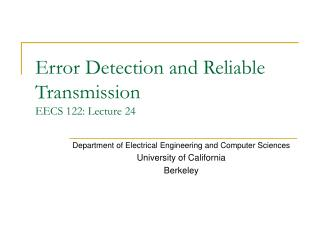Error Detection and Reliable Transmission EECS 122: Lecture 24

# Error Detection and Reliable Transmission EECS 122: Lecture 24 - PowerPoint PPT Presentation

Error Detection and Reliable Transmission EECS 122: Lecture 24. Department of Electrical Engineering and Computer Sciences University of California Berkeley. Today. Focus on a Link Layer function Bits need to be sent over a link in frames Bits may be corrupted in transitI am the owner, or an agent authorized to act on behalf of the owner, of the copyrighted work described.
Download Presentation## Error Detection and Reliable Transmission EECS 122: Lecture 24

An Image/Link below is provided (as is) to download presentation

Download Policy: Content on the Website is provided to you AS IS for your information and personal use and may not be sold / licensed / shared on other websites without getting consent from its author.While downloading, if for some reason you are not able to download a presentation, the publisher may have deleted the file from their server.

- - - - - - - - - - - - - - - - - - - - - - - - - - E N D - - - - - - - - - - - - - - - - - - - - - - - - - -
Presentation Transcript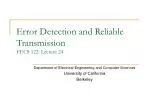### Error Detection and Reliable TransmissionEECS 122: Lecture 24

Department of Electrical Engineering and Computer Sciences

University of California

Berkeley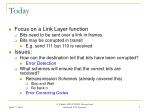Today
• Focus on a Link Layer function
• Bits need to be sent over a link in frames
• Bits may be corrupted in transit
• E.g. send 111 but 110 is received
• Issues:
• How can the destination tell that bits have been corrupted?
• Error Detection
• What schemes will ensure that the correct bits are received?
• Retransmission Schemes (already covered this)
• Stop and Wait
• Go back n
• Error Correcting Codes

A. Parekh, EE122 S2003. Revised and enhanced F'02 Lectures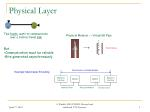Two hosts, want to communicate

over a bidirectional link

Physical Layer

Physical Medium → Virtual Bit Pipe

101101

1

XXX

• But…
• Communication must be reliable
• Bits generated asynchronously

Synchronous

Synchronous unreliable bit pipe

Example: Manchester Encoding

RS232-CInterface

Modem

Modem

RS232-CInterface

Interface wires

Physical Medium

Interface wires

A. Parekh, EE122 S2003. Revised and enhanced F'02 Lectures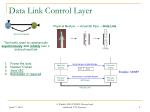10101

1

Frames

Two hosts, want to communicate

asynchronously and reliably over a

Data Link Control Layer

Physical Medium → Virtual Bit Pipe → Data Link

101101

1

XXX

• Frame the data
• Number frames
• Send CRC
• Retransmit if required

Asynchronous reliable bit pipe

Control

Control

FH

Data

Example: SONET

Synchronous unreliable bit pipe

PhysicalInterface

PhysicalInterface

Bit Stream

A. Parekh, EE122 S2003. Revised and enhanced F'02 Lectures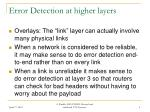Error Detection at higher layers
• Overlays: The “link” layer can actually involve many physical links
• When a network is considered to be reliable, it may make sense to do error detection end-to-end rather than on every link
• When a link is unreliable it makes sense to do error detection at layer 3 so that routers can check for bad headers without having to worry about the payload

A. Parekh, EE122 S2003. Revised and enhanced F'02 Lectures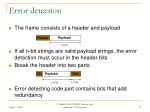Error detection
• The frame consists of a header and payload
• If all n-bit strings are valid payload strings, the error detection must occur in the header bits
• Break the header into two parts
• Error detecting code part contains bits that add redundancy

n bits

FH

EDC

k bits

n bits

A. Parekh, EE122 S2003. Revised and enhanced F'02 Lectures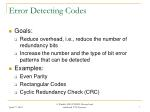Error Detecting Codes
• Goals:
• Reduce overhead, i.e., reduce the number of redundancy bits
• Increase the number and the type of bit error patterns that can be detected
• Examples:
• Even Parity
• Rectangular Codes
• Cyclic Redundancy Check (CRC)

A. Parekh, EE122 S2003. Revised and enhanced F'02 Lectures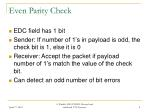Even Parity Check
• EDC field has 1 bit
• Sender: If number of 1’s in payload is odd, the check bit is 1, else it is 0
• Receiver: Accept the packet if payload number of 1’s match the value of the check bit.
• Can detect an odd number of bit errors

A. Parekh, EE122 S2003. Revised and enhanced F'02 Lectures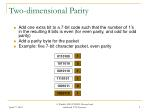Two-dimensional Parity
• Add one extra bit to a 7-bit code such that the number of 1’s in the resulting 8 bits is even (for even parity, and odd for odd parity)
• Add a parity byte for the packet
• Example: five 7-bit character packet, even parity

0110100

1

1011010

0

0010110

1

1110101

1

1001011

0

1000110

1

A. Parekh, EE122 S2003. Revised and enhanced F'02 Lectures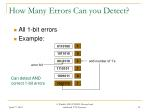How Many Errors Can you Detect?
• All 1-bit errors
• Example:

0110100

1

1011010

0

0000110

odd number of 1’s

1

error bit

1110101

1

1001011

0

Can detect AND

correct 1-bit errors

1000110

1

A. Parekh, EE122 S2003. Revised and enhanced F'02 LecturesHow Many Errors Can you Detect?
• All 2-bit errors
• Example:

0110100

1

1011010

0

0000111

1

error bits

1110101

1

1001011

0

1000110

1

odd number of 1’s on column

A. Parekh, EE122 S2003. Revised and enhanced F'02 LecturesHow Many Errors Can you Detect?
• All 3-bit errors
• Example:

0110100

1

1011010

0

0000111

1

error bits

1100101

1

1001011

0

1000110

1

odd number of 1’s on column

A. Parekh, EE122 S2003. Revised and enhanced F'02 LecturesHow Many Errors Can you Detect?
• Most 4-bit errors
• Example of 4-bit error that is not detected:

0110100

1

1011010

0

0000111

1

error bits

1100100

1

1001011

0

1000110

1

A. Parekh, EE122 S2003. Revised and enhanced F'02 LecturesBurst Errors
• Often errors occur in bursts, i.e. successive bits are corrupted.
• Many detecting/correcting schemes fail
• Use an interleaving trick…
• Suppose we 4 code words to send
• 0101001,0000000,111111,1010101
• Send position 1 of each codeword, then position 2 of each codeword etc.
• 0011 1010 0011 1010 0010 0010 1011
• A burst of 4 errors looks like 4 single bit errors to each of the code words!

A. Parekh, EE122 S2003. Revised and enhanced F'02 LecturesCyclic Redundancy Check Codes (CRC)
• Very popular error detection code
• Efficient Implementations in HW and SW
• Represent the payload as a polynomial
• The EDC bits are generated by dividing the payload polynomial by another special polynomial called the generator.
• The generator can be picked to ensure that a variety of different errors are detected.

A. Parekh, EE122 S2003. Revised and enhanced F'02 LecturesCyclic Redundancy Check (CRC)
• Represent a n-bit payload by an polynomial P(x)
• E.g., 10101101  P(x) = x7 + x5 + x3 + x2 + x0
• Choose a generator k-degree polynomial G(x)
• Compute remainder R(x) of P(x)*xk / G(x)
• Note that P(x)*xk represents the the payload shifted to the left by k places
• E.g. If P(x)=x7 + x5 + x3 + x2 + x0 and k=2 then
• P(x)*xk = x9 + x7 + x5 + x4 + x2  1010110100
• The EDC bits are derived from -R(x)
• A-B in binary is a bitwise XOR
• E.g. If k=2 and R(x)=1 then EDC bits are 00 – 01 = 01

A. Parekh, EE122 S2003. Revised and enhanced F'02 LecturesCRC
• T(x) = M(x)*xk - R(x)
• T(x) is divisible by C(x)
• First n coefficients of T(x) represent M(x)
• E.g. If P(x)=x7 + x5 + x3 + x2 + x0 ,R(x)=x+1, k=2 then T(x) = x9 + x7 + x5 + x4 + x2 +1
• Sender:
• Compute and send T(x), i.e., the coefficients of T(x)
• Let T’(x) is what he gets
• If C(x) divides T’(x)  no errors; otherwise errors

A. Parekh, EE122 S2003. Revised and enhanced F'02 LecturesComputing R(x)
• Compute the reminder R(x) of P(x)*xk / G(x)
• T(x) = P(x)*xk - R(x)
• Example: send packet 110111, assume G(x) = 101
• k = 2, P(x)*xK  11011100
• Compute R(x)
• T(x) = P(x)*xk - R(x)  11011100 xor 1 = 11011101

101) 11011100

101

111

101

101

101

100

101

1

R(x)

A. Parekh, EE122 S2003. Revised and enhanced F'02 LecturesCheck at the receiver

101) 11011101

101

111

101

101

101

101

101

0

No errors

A. Parekh, EE122 S2003. Revised and enhanced F'02 LecturesProperties of CRCs
• Detect all single-bit errors if coefficients of xk and x0 of G(x) are one
• Detect all double-bit errors, if G(x) has a factor with at least three terms
• Detect all number of odd errors, if G(x) contains factor (x+1)
• Detect all burst of errors smaller than k bits

A. Parekh, EE122 S2003. Revised and enhanced F'02 LecturesWhat’s going on?
• Think of the combination of the n payload bits and the k EDC bits as being a n+k bit codeword
• For any error correcting scheme, not all n+k bit strings will be valid code words
• Errors can be detected if and only if the received string is not a valid codeword
• Example: Even parity check only detects an odd number of bit errors

A. Parekh, EE122 S2003. Revised and enhanced F'02 LecturesHamming Distance
• Given codewords A and B, the Hamming distance between them is the number of bits in A that need to be flipped to turn it into B
• Example H(011101,000000)=4
• If all codewords are at least d Hamming distance apart, then up to d-1 bit errors can be detected

A. Parekh, EE122 S2003. Revised and enhanced F'02 LecturesError Correction
• If all the codewords are at least a hamming distance of 2E+1 apart then up to E bit errors can be detected
• Just pick the codeword closest to the one received!
• How many bits of EDC are required to correct d errors when there are n bits in the payload?
• Example: d=1: Suppose n=3. Then any payload can be transformed into 3 other payload strings (000 into 001, 010 or 100).
• Need at least two bits in the ECD to differentiate between 4 possibilities
• In general need at least k ≥ log2(n+1) bits
• A scheme that is optimal is called a perfect parity code

A. Parekh, EE122 S2003. Revised and enhanced F'02 LecturesPerfect Parity Codes
• Consider a code word of n+k bits
• b1 b2 b3 b4 b5 b6 b7 b8 b9 b10 b11…
• Parity bits are in positions 20, 21, 22 ,23 ,24…
• b1b2b3 b4b5 b6 b7 b8b9 b10 b11…
• A parity bit in position 2h, checks all data bits bp such that if you write out p in binary, the hth place is a one.
• Example: b1 is a parity bit. It is applied over all data bits bp such that the zeroth position of p is a one, i.e. p is odd

A. Parekh, EE122 S2003. Revised and enhanced F'02 LecturesA (7,4)-Perfect Parity Code
• n=4, k=3
• Since log(n+1)= 2.322, a code with k=3 that corrects one error is “perfect”
• Supposing the 6th bit is corrupted in a codeword. The binary rep of 6 is 110, so the parity bits at positions 2 and 4 would fail. There is no other case when these two bits would fail together
• Note 2+4 is 6
• Suppose the 5th bit fails. 5101 so the parity bits at positions 1 and 4 would fail
• Note 1+4 is 5
• Suppose the 4th bit is corrupted. 4 100 so the parity bit at position 4 would fail
• Corrupted Parity bits can be identified as well

A. Parekh, EE122 S2003. Revised and enhanced F'02 LecturesReliable Transmission
• Problem: obtain correct information once errors are detected
• Solutions:
• Use error correction codes (e.g. perfect parity codes)
• Use retransmission (we have studied this already)
• Algorithmic challenges:
• Achieve high link utilization, and low overhead

A. Parekh, EE122 S2003. Revised and enhanced F'02 LecturesError correction or Retransmission?
• Error Correction requires a lot of redundancy
• Wasteful if errors are unlikely
• Retransmission strategies are more popular
• As links get reliable this is just done at the transport layer
• Error correction is useful when retransmission is costly (satellite links, multicast)

A. Parekh, EE122 S2003. Revised and enhanced F'02 Lectures